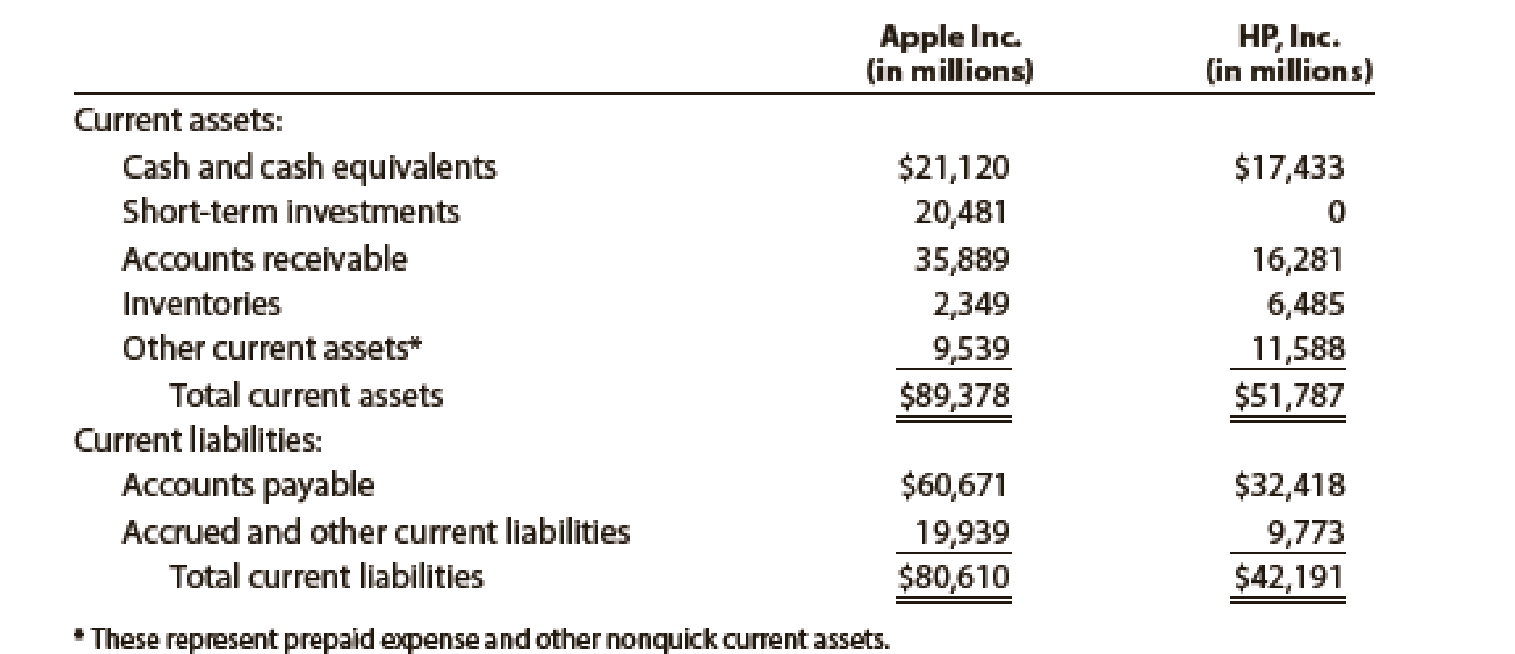# Quick ratio The current assets and current liabilities for Apple Inc. and HP, Inc., are as follows at the end of a recent fiscal period: a. Determine the quick ratio for both companies. Round to one decimal place. b. Interpret the quick ratio difference between the two companies.### Financial Accounting

15th Edition
Carl Warren + 2 others
Publisher: Cengage Learning
ISBN: 9781337272124

#### Solutions

Chapter
Section### Financial Accounting

15th Edition
Carl Warren + 2 others
Publisher: Cengage Learning
ISBN: 9781337272124
Chapter 11, Problem 24E
Textbook Problem
3 views

## Quick ratioThe current assets and current liabilities for Apple Inc. and HP, Inc., are as follows at the end of a recent fiscal period:a. Determine the quick ratio for both companies. Round to one decimal place. b. Interpret the quick ratio difference between the two companies.

a.

To determine

Calculate Quick ratio for Incorporation A and Incorporation H.

### Explanation of Solution

Quick ratio: Quick ratio, also called as acid-test ratio, denotes that this ratio is a more rigorous test of solvency than the current ratio. It is determined by dividing quick assets and current liabilities. The acceptable quick ratio is 0.90 to 1.00. Use the following formula to determine the quick ratio:

Quick Ratio=Quick assetsCurrentliabilities

Quick Assets: Quick assets are those assets that are most liquid. The examples of quick assets include cash and bank balances, temporary investments, and accounts receivable.

Current liabilities: Current liability is a kind of liability or the obligation of the business towards the creditors, in which the business is required to pay the creditors, within a period of one year or one operating cycle of the business, whichever is longer. The examples of current liabilities include Accounts payable, Salaries and Wages payable, Interest payable, Income Tax payable.

Determine Quick ratio for Incorporation A, if Cash is given as $21,120 million, short-term investment is given as$20,481million, accounts receivable is given as $35,889 million, and current liabilities is given as$80,610 million

b.

To determine

Interpret Quick ratio between Incorporation A and Incorporation H.

### Still sussing out bartleby?

Check out a sample textbook solution.

See a sample solution

#### The Solution to Your Study Problems

Bartleby provides explanations to thousands of textbook problems written by our experts, many with advanced degrees!

Get Started